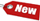Popular Accounting TopicsAccounting for Merchandising ActivitiesDebits and Credits (Double Entry Accounting)Time Value of Money & Present/Future ValuesComplex Debt & Equity InstrumentsCommon Stock & Shareholder's EquityAccounting & Finance RatiosValuing Common StockCorporate Income TaxesLower of Cost or Market (LCM) & Inventory ValuationChart of Accounts & BookkeepingBonds Payable & Long Term LiabilitiesCapital Assets What category of browser are you on this website? Accounting student (homework help) Finance professor (university research) Accounting manager (at work) Other Explore Careers in Accounting & Finance®Visit our section on Careers in Accounting & Finance to explore vast opportunities in this industry.

# Compound Interest

Interest earned on both the initial principal and the interest reinvested from prior periods. Go here to learn more about compound interest.

Compound Interest Homework ProblemConsider you found an investment that pays 15% annual interest, which sounds fantastic to you! This makes you invest \$1000 in to it, how much will you have in 3 years? How much will you have in 7 years? And at the end of 7 years, how much interest will you have earned, and how much of it will be compounding interest?
Note: We recommend you try this problem without looking at the solution below first, however if you get stuck, feel free to take a peak!

 a) Future Value = \$1 x (1 + r)t = =\$1 x (1 + 0.15)3 = \$1.153 = 1.520875 x \$1000 = \$1,520.875 b) Future Value = \$1 x (1 + r)t = =\$1 x (1 + 0.15)7 = \$1.157 = 2.66002 x \$1000 = \$2,660.02

You invested \$1000 x 15% = \$150 simple interest each year, thus total simple interest in 7 years = \$150 x 7 = \$1,050

 Compound Interest = Total Interest – Simple Interest Compound Interest = \$1,660.02 -\$1050 Compound Interest = \$610.02